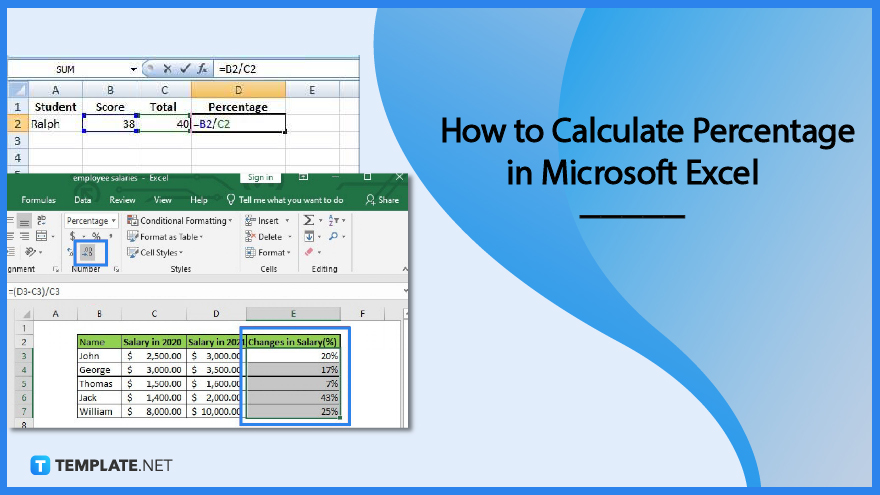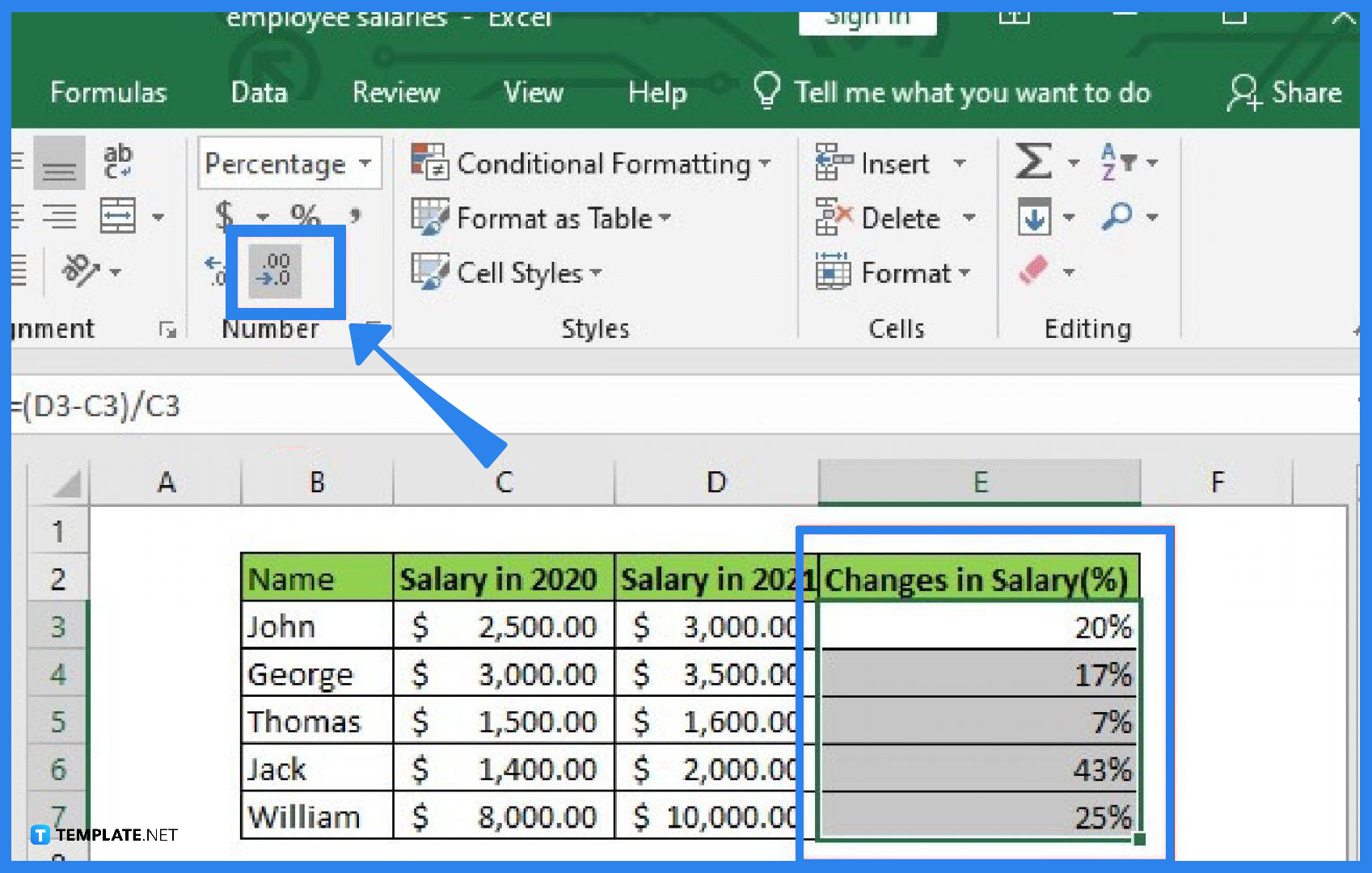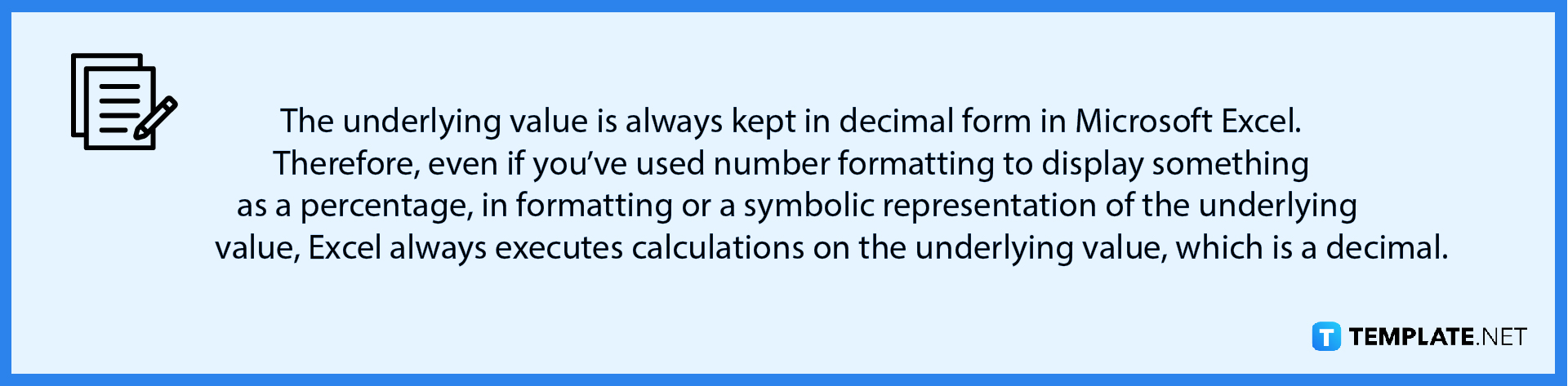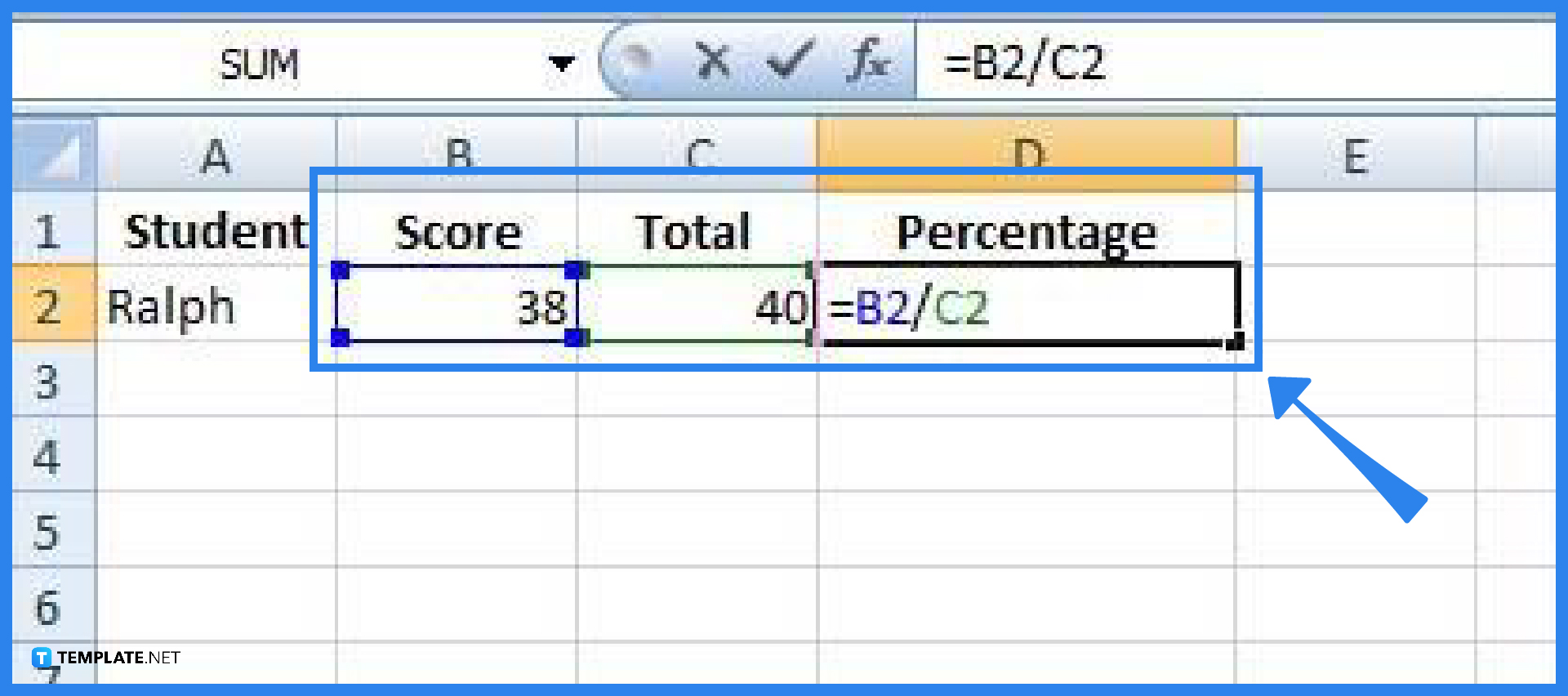# How to Calculate Percentage in Microsoft Excel

Calculating percentages in Microsoft Excel is quite easy as the software provides a bunch of ways to do the job. Excel will provide you with basic formulas to determine the percentage of a total or the difference between two values.## How to Calculate Percentage in Microsoft Excel

Microsoft Excel provides you with different ways to calculate percentages such as formulas and formatting. Calculating the values in percentage takes only two steps in Microsoft Excel.

• ### Step 1: Format the Values as PercentagesApplying the percentage format to the cells will allow you to display a number in Microsoft Excel as a percentage. To do this, simply pick the cells to format, and then on the Home tab of the ribbon, click the Percent Style (%) button in the Number group. The decimal place can then be adjusted to increase or decrease as necessary.• ### Step 2: Calculate the Values in Percentage Using FormulasAs with any formula in Microsoft Excel, you must first enter an equal sign (=) in the cell where you want the result before entering the remaining formula variables. The fundamental formula for determining percentages is “=part/total”. As for the example shown in the above image, score/total = grade in %.

## FAQs

### How to compute the percentage of a total in Microsoft Excel?

Click on any blank cell in the spreadsheet, type the values to calculate in the form of =part/total (for example, you can type =42/50 and press Enter and you will get a result of 0.84), select the cell that contains the result, then go to the Home tab, and click on the % symbol (the result would change into a percentage form, which is 84.00%); you can also change the number of decimal places of the result by selecting the Increase Decimal or Decrease Decimal symbols.

### How to calculate the percentage of increase between two numbers?

Click on any spreadsheet cell, type the values to calculate in the form of =(total-part)/part (for example, you can type =(2500-2342)/2342, press Enter, and you will get a result of 0.06746), select the cell that contains the result, then go to the Home tab, and click on the % symbol (the result will change into a percentage form, which is 6.75%); you can also change the number of decimal places in the result by choosing the Increase Decimal or Decrease Decimal symbols.

### How do I change an amount by a percentage in Microsoft Excel?

In the first cell, enter the amount you wish to change by a percentage (place \$200, for example, in cell A1); then, select the second cell next to the first selected cell, right-click on that cell and select Format Cells, then select Percentage, click OK, and in that second cell, enter the percentage you want to subtract from the initial amount (place 17%, for example, in B1), and choose the third cell, inside of which you wish to show the changed amount and write the formula in the form of =initial amount*(1-given percentage); as for the given example, you will write the formula as “=A1*(1-B1)”, and get the result of \$166 after pressing Enter.